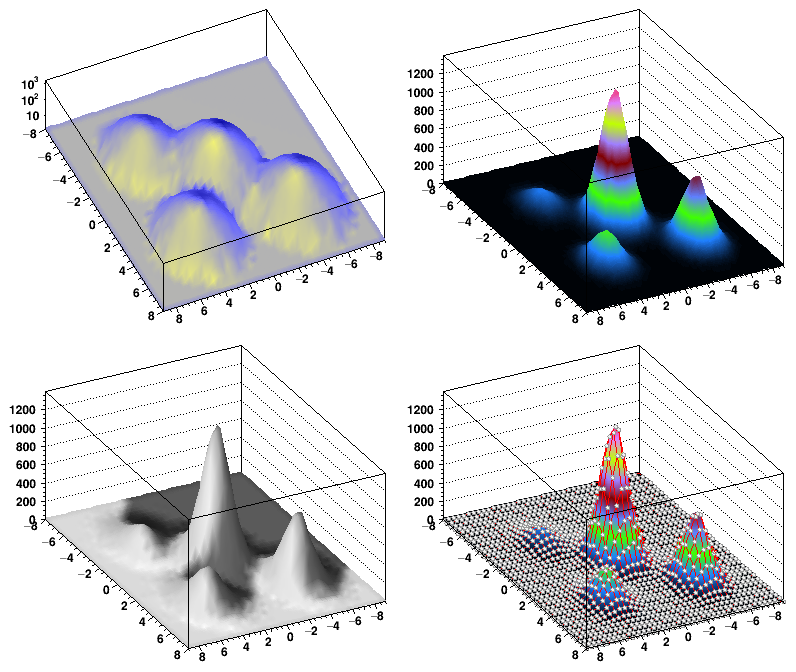# spectrumpainter¶

Examples showing how to use TSpectrum2Painter (the SPEC option)

Author: Olivier Couet, Miroslav Morhac
This notebook tutorial was automatically generated with ROOTBOOK-izer from the macro found in the ROOT repository on Sunday, October 02, 2022 at 09:38 AM.

In :
TH2 *h2 = new TH2F("h2","h2",40,-8,8,40,-9,9);
Float_t px, py;
for (Int_t i = 0; i < 50000; i++) {
gRandom->Rannor(px,py);
h2->Fill(px,py);
h2->Fill(px+4,py-4,0.5);
h2->Fill(px+4,py+4,0.25);
h2->Fill(px-3,py+4,0.125);
}

TCanvas *c1 = new TCanvas("c1","Illustration of 2D graphics",10,10,800,700);
c1->Divide(2,2);

c1->cd(1);
h2->Draw("SPEC dm(2,10) zs(1)");
c1->cd(2);
h2->Draw("SPEC dm(1,10) pa(2,1,1) ci(1,4,8) a(15,45,0)");
c1->cd(3);
h2->Draw("SPEC dm(2,10) pa(1,1,1) ci(1,1,1) a(15,45,0) s(1,1)");
c1->cd(4);
h2->Draw("SPEC dm(1,10) pa(2,1,1) ci(1,4,8) a(15,45,0) cm(1,15,4,4,1) cg(1,2)");


Draw all canvases

In :
gROOT->GetListOfCanvases()->Draw()